How to

# How to do an Index Match formula

Index match formulas fully explained

Index match formulas fully explained

Index match formulas fully explained

Aug 12, 2022

Aug 12, 2022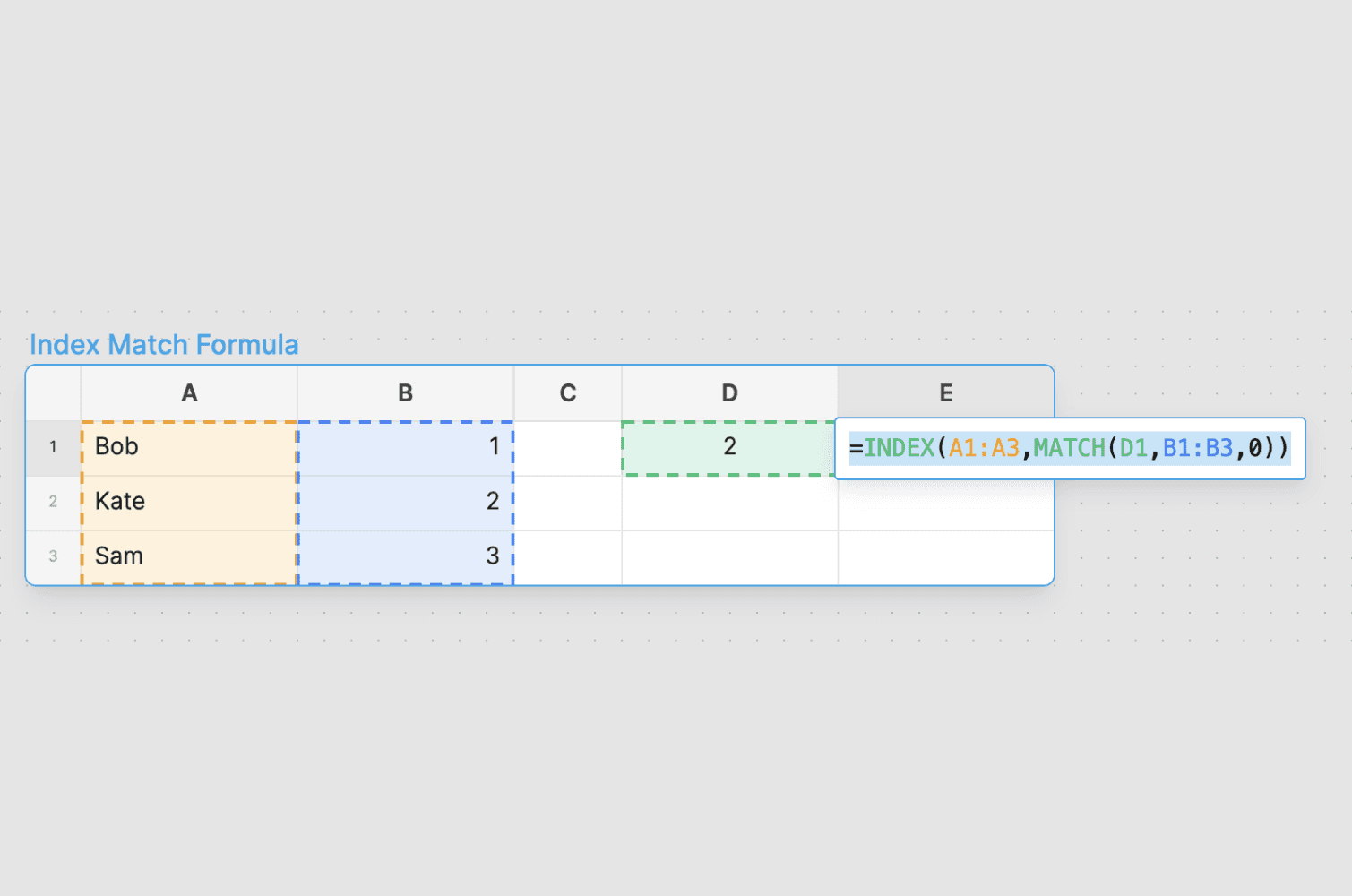# How to do an Index Match formula

Say you had a big list of incoming 1st year investment banking salaries, such as the below (data from WallStreetOasis.com):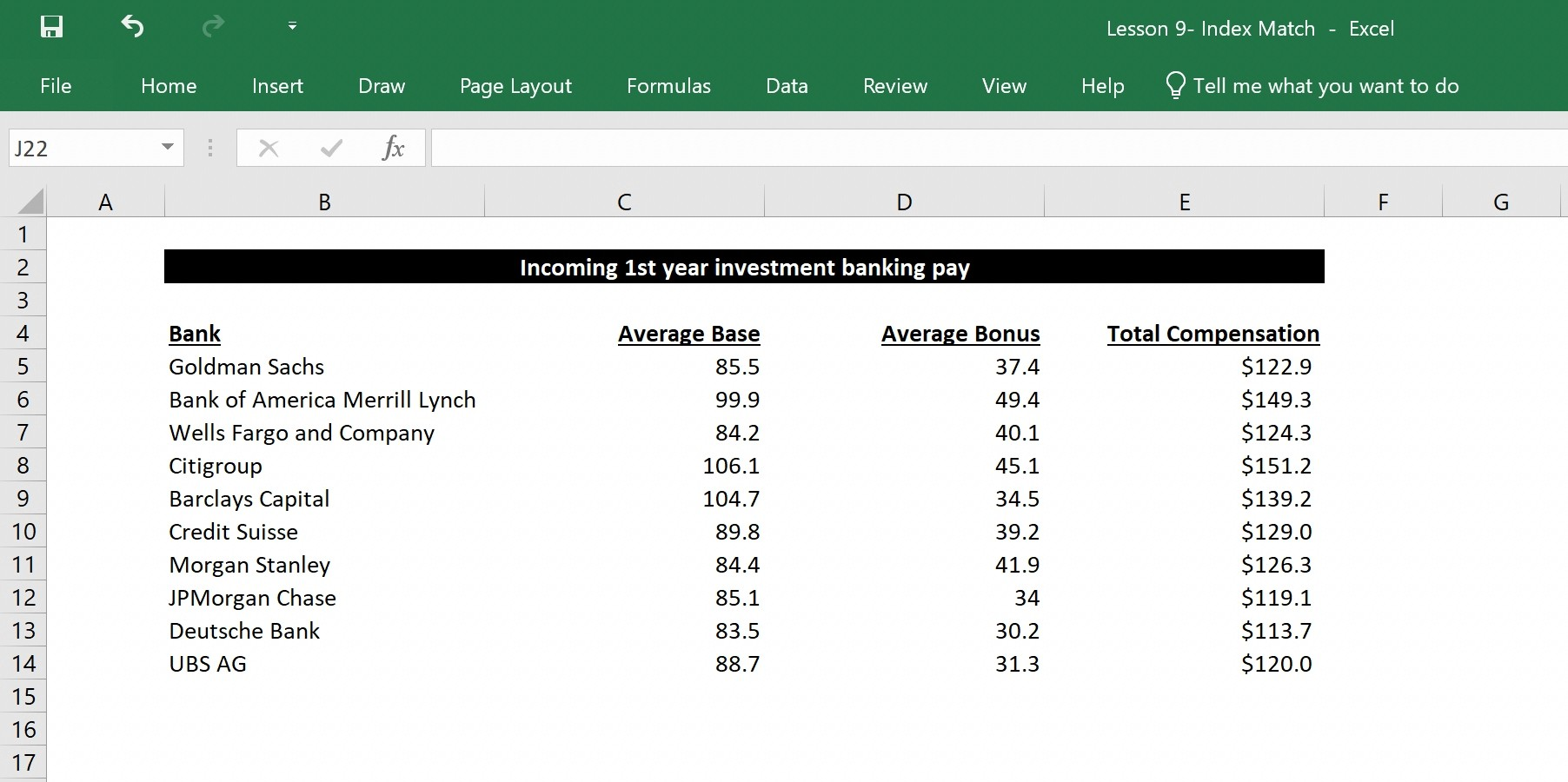Now let's say you only wanted to see the total pay of the banks that you received an offer from. It may not be too hard to just look at the list and delete the banks you got rejected from or manually type in each of the banks for now, but imagine you had to do this for a list of 10,000+ entries. This is when you can use Index Match to help automate your work and prevent errors. There is also the VLOOKUP and HLOOKUP functions, but you should just learn the INDEX MATCH formula. The reasons are:

(1) it's more flexible, because you can search both columns and rows

(2) it's easier to drag and reuse the formula

(3) it can insert columns or rows in the look up array

(4) it can search left to right or right to left

(5) it's less taxing for Excel to calculate (was told by someone wiser than me, but have not verified). But aside from these reasons, from a practical standpoint, in all my years of finance, INDEX MATCH could do everything VLOOKUP could, but the opposite wasn't true.

So with the above example, if you had a list of the banks you had a job offer for, you could easily create a formula to look up what the compensation is for each of them. INDEX MATCH would help you fill in the red highlighted cells programatically. Even better, you would only have to fill in cell B20 and then you could drag the formulas to the right with CTRL+R and down with CTRL+D.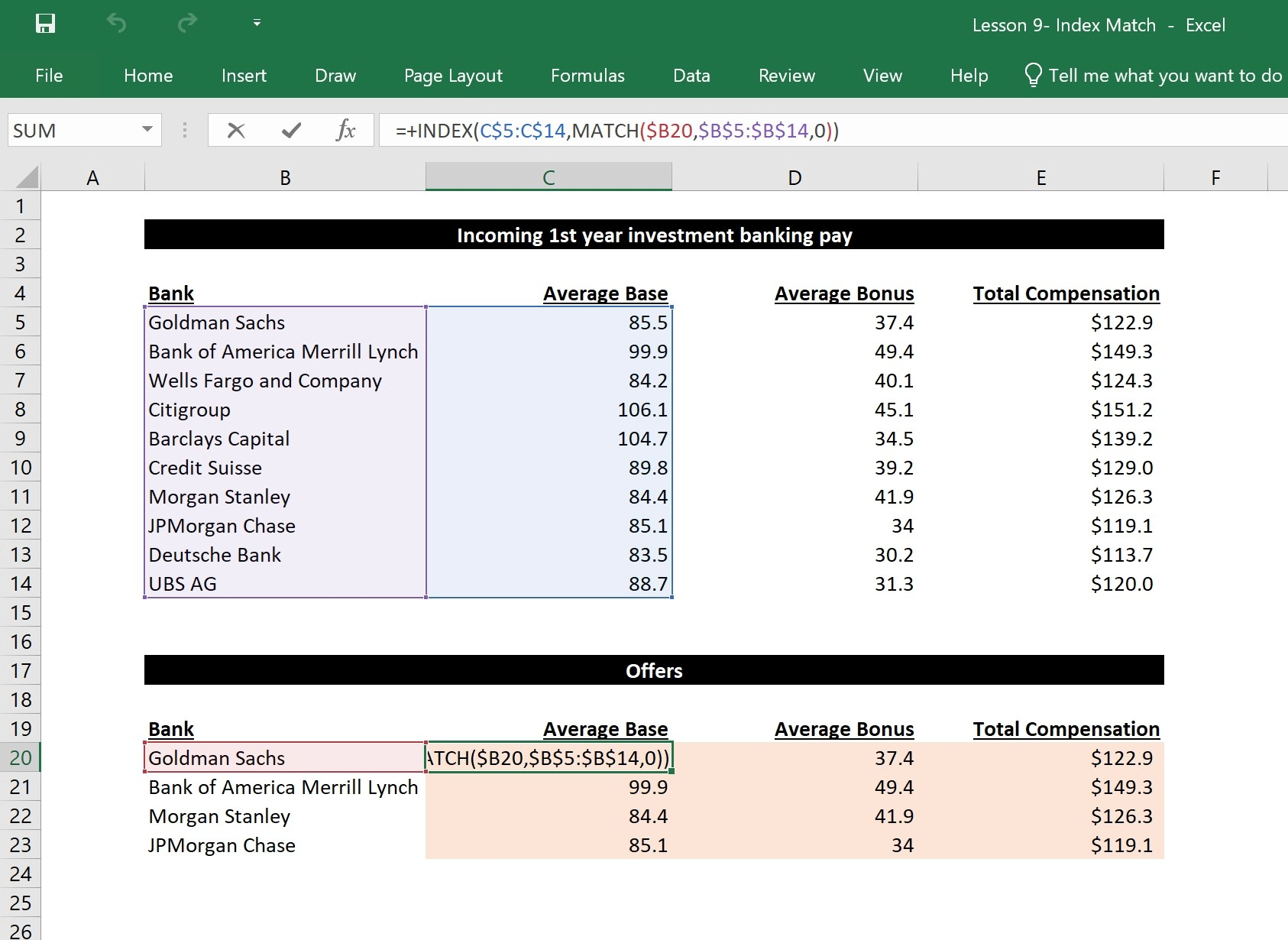When you look at the complex formula, the simple breakdown is that you want to return an answer by matching up something unique in two different tables. It's a hard concept to grasp in text, so hopefully the visual below helps along with my attempt to explain it.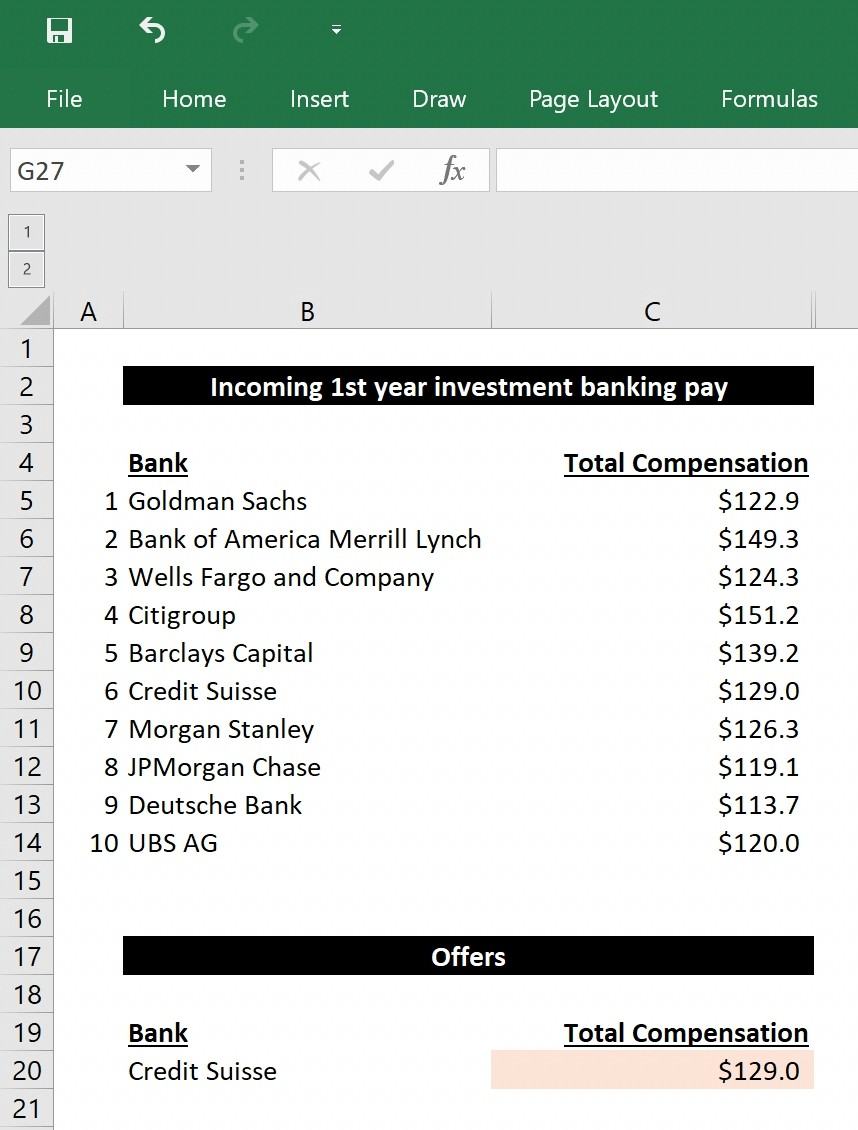INDEX MATCH is a combination of two distinct functions. The way the INDEX formula works is that it returns a value based on a location in a range. In our case (above) Goldman Sachs is 1, Bank of America Merrill Lynch is 2, Wells Fargo and Company is 3, so on and so forth. So if you wanted to return Credit Suisse, you could just type in =+INDEX(B5:B14,6).

Now the MATCH formula can help you return a number based on a position in a range. So if I wrote a formula =+MATCH("Credit Suisse",B5:B14,0), it would give us the number 6 because Credit Suisse is the sixth item in the range. Note that you need the extra '0' at the end to signify that you want an exact match (something you can just memorize).

Now if you combine those two concepts, you can now get the 6th bank's compensation by indexing the total compensation column (you want to return an item in this range) and matching it to the bank that you want (this will give you the location of the bank, which corresponds to the location of where the total compensation is). See below for an example, which will give you the answer of \$129.0 since its the 6th item on the compensation list and Credit Suisse is the 6th bank on the bank list.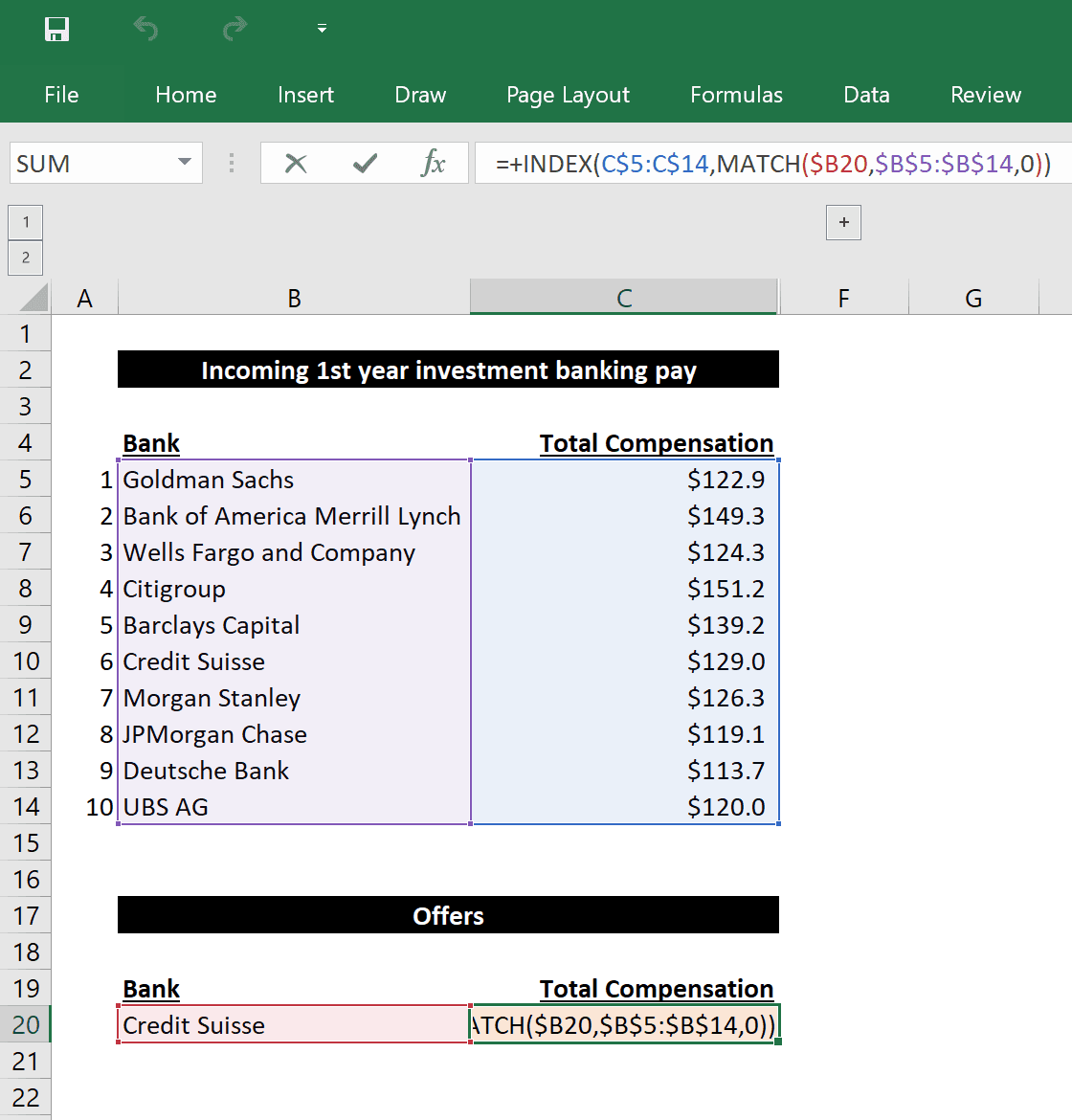Notice that in the example above, the first range is only one column wide and therefore you only need one match. However INDEX MATCH can also handle entire arrays. In those cases, you must match a row as well, in the same manner that you matched the column in the prior example.

While this is a good intro to the INDEX MATCH formula, this is another tool that you will learn more by doing. It's a formula that you will use all the time for financial analysis, so get familiar with it!

# How to do an Index Match formula

Say you had a big list of incoming 1st year investment banking salaries, such as the below (data from WallStreetOasis.com):Now let's say you only wanted to see the total pay of the banks that you received an offer from. It may not be too hard to just look at the list and delete the banks you got rejected from or manually type in each of the banks for now, but imagine you had to do this for a list of 10,000+ entries. This is when you can use Index Match to help automate your work and prevent errors. There is also the VLOOKUP and HLOOKUP functions, but you should just learn the INDEX MATCH formula. The reasons are:

(1) it's more flexible, because you can search both columns and rows

(2) it's easier to drag and reuse the formula

(3) it can insert columns or rows in the look up array

(4) it can search left to right or right to left

(5) it's less taxing for Excel to calculate (was told by someone wiser than me, but have not verified). But aside from these reasons, from a practical standpoint, in all my years of finance, INDEX MATCH could do everything VLOOKUP could, but the opposite wasn't true.

So with the above example, if you had a list of the banks you had a job offer for, you could easily create a formula to look up what the compensation is for each of them. INDEX MATCH would help you fill in the red highlighted cells programatically. Even better, you would only have to fill in cell B20 and then you could drag the formulas to the right with CTRL+R and down with CTRL+D.When you look at the complex formula, the simple breakdown is that you want to return an answer by matching up something unique in two different tables. It's a hard concept to grasp in text, so hopefully the visual below helps along with my attempt to explain it.INDEX MATCH is a combination of two distinct functions. The way the INDEX formula works is that it returns a value based on a location in a range. In our case (above) Goldman Sachs is 1, Bank of America Merrill Lynch is 2, Wells Fargo and Company is 3, so on and so forth. So if you wanted to return Credit Suisse, you could just type in =+INDEX(B5:B14,6).

Now the MATCH formula can help you return a number based on a position in a range. So if I wrote a formula =+MATCH("Credit Suisse",B5:B14,0), it would give us the number 6 because Credit Suisse is the sixth item in the range. Note that you need the extra '0' at the end to signify that you want an exact match (something you can just memorize).

Now if you combine those two concepts, you can now get the 6th bank's compensation by indexing the total compensation column (you want to return an item in this range) and matching it to the bank that you want (this will give you the location of the bank, which corresponds to the location of where the total compensation is). See below for an example, which will give you the answer of \$129.0 since its the 6th item on the compensation list and Credit Suisse is the 6th bank on the bank list.Notice that in the example above, the first range is only one column wide and therefore you only need one match. However INDEX MATCH can also handle entire arrays. In those cases, you must match a row as well, in the same manner that you matched the column in the prior example.

While this is a good intro to the INDEX MATCH formula, this is another tool that you will learn more by doing. It's a formula that you will use all the time for financial analysis, so get familiar with it!